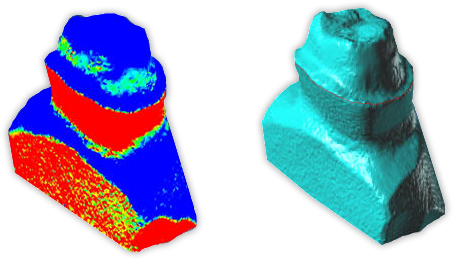Draft Angle Contouring
Windows only

## Problem

Rhino’s draft angle analysis is very useful. However, it would be great it it could create contour curves at specific angles. For example:Notice the red curve on the right-hand image above. This is what you would like to automate. Is there an Rhino function that will help do this?

## Solution

There is not an function that will help you do this. But, it is possible to write your own tool.

Draft angle analysis works by calculating the angles between mesh vertex normals and the unit normal (in most cases this is the world z-axis). It is possible to perform this calculation from a plugin command. From these angles, it is possible to determine whether or not a contour line would pass through a mesh vertex or if it would cross between two mesh vertices.

## Sample

The follow sample code demonstrates this process:

CRhinoCommand::result CCommandTest::RunCommand( const CRhinoCommandContext& context )
{
// Pick a mesh object
CRhinoGetObject go;
go.SetCommandPrompt( L"Select mesh for draft angle contour" );
go.SetGeometryFilter( CRhinoGetObject::mesh_object );
go.GetObjects( 1, 1 );
if( go.CommandResult() != success )
return go.CommandResult();

const ON_Mesh* pMesh = go.Object(0).Mesh();
if( 0 == pMesh )
return failure;

// Copy mesh so we can tweak it if necessary
ON_Mesh mesh( *pMesh );

// To make our life easy, convert all quads to triangles.

// For draft angle analysis, mesh must have vertex normals.
if( !mesh.HasVertexNormals() )
{
if( !mesh.ComputeVertexNormals() )
return failure;
}

// Specify a draft angle
CRhinoGetNumber gn;
gn.SetCommandPrompt( L"Draft angle" );
gn.SetDefaultNumber( m_angle );
gn.SetLowerLimit( 0.0, TRUE );
gn.SetUpperLimit( 90.0, TRUE );
gn.GetNumber();
if( gn.CommandResult() != success )
return gn.CommandResult();

m_angle = gn.Number(); // degrees

//////////////////////////////////////////////////////////////

double A = m_angle * ( ON_PI / 180.0 ); // alpha
ON_3dVector D = ON_zaxis; // unit normal
int fvcnt = 3; // triangles

// Process each mesh face
int fi, i, j;
for( fi = 0; fi < mesh.m_F.Count(); fi++ )
{
const ON_MeshFace& f = mesh.m_F[fi];

// For each face vertex, calculate the draft angle
double d, a;
for( i = fvcnt - 1; i >= 0; i-- )
{
ON_3dVector N = mesh.m_N[f.vi[i]];
d[i] = RHINO_CLAMP( N * D, -1.0, 1.0 );
a[i] = acos(d[i]) - A;
}

// Determine if any of the angles meet our criteria.
// If so, calculate a point on an edge at that angle.
int P_count = 0;
ON_3dPoint P;

for( i = 0; i < fvcnt; i++ )
{
j = (i + 1) % fvcnt;

// If zero, then draft angle point passes through vertex
if( a[i] == 0 )
P[P_count++] = mesh.m_V[f.vi[i]];

// See if draft angle point crosses the edge between two vertices
else if( a[i] * a[j] < 0.0 )
{
double t = a[i] / (a[i] - a[j]);
P[P_count++] = ((1 - t) * mesh.m_V[f.vi[i]]) + (t * mesh.m_V[f.vi[j]]);
}
}

// If we have calculated enough points, create some geometry
if( P_count == 2 )